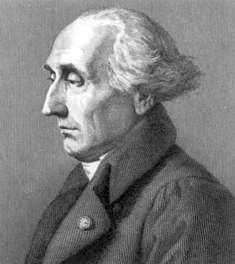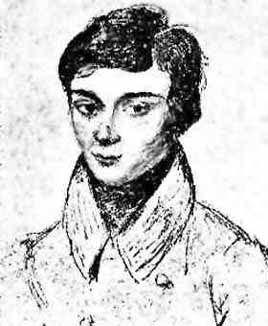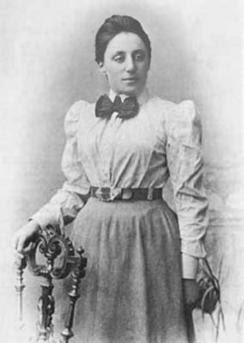### Notes on Emmy Noether and Group TheoryJust a few quick notes on historical trails I'm finding as I study group theory and Emmy Noether's theorem. The people involved are a bit of a group themselves. The first person I found is Lagrange. He introduced the Langrangian, one of the key concepts of analytical mechanics. It's used today, well, everywhere, from plain old mechanics to quantum mechanics, to quantum field theory. He also laid some of the foundations of group theory working on permutation groups and their use in solving polynomials.That brings us to Évariste Galois. Galois read Lagrange's papers at the age of 15. He later went on to develop Galois theory. Galois theory relates permutation groups of the roots of polynomials to their solvability.Sophus Lie developed Lie groups, provide a framework that is similar to Galois theory for studying the symmetries of differential equations.And that brings us to Emmy Noether. Her paper on "Invariant Variation Problems" applied Lie's work to variational calculus revealing conservation laws applied to Lagrangians.

### Cool Math Tricks: Deriving the Divergence, (Del or Nabla) into New (Cylindrical) Coordinate Systems

The following is a pretty lengthy procedure, but converting the divergence, (nabla, del) operator between coordinate systems comes up pretty often. While there are tables for converting between common coordinate systems, there seem to be fewer explanations of the procedure for deriving the conversion, so here goes!

What do we actually want?

To convert the Cartesian nabla

to the nabla for another coordinate system, say… cylindrical coordinates.

What we’ll need:

1. The Cartesian Nabla:

2. A set of equations relating the Cartesian coordinates to cylindrical coordinates:

3. A set of equations relating the Cartesian basis vectors to the basis vectors of the new coordinate system:

How to do it:

Use the chain rule for differentiation to convert the derivatives with respect to the Cartesian variables to derivatives with respect to the cylindrical variables.

The chain rule can be used to convert a differential operator in terms of one variable into a series of differential operators in terms of othe…

### The Valentine's Day Magnetic Monopole

There's an assymetry to the form of the two Maxwell's equations shown in picture 1.  While the divergence of the electric field is proportional to the electric charge density at a given point, the divergence of the magnetic field is equal to zero.  This is typically explained in the following way.  While we know that electrons, the fundamental electric charge carriers exist, evidence seems to indicate that magnetic monopoles, the particles that would carry magnetic 'charge', either don't exist, or, the energies required to create them are so high that they are exceedingly rare.  That doesn't stop us from looking for them though!

Keeping with the theme of Fairbank and his academic progeny over the semester break, today's post is about the discovery of a magnetic monopole candidate event by one of the Fairbank's graduate students, Blas Cabrera.  Cabrera was utilizing a loop type of magnetic monopole detector.  Its operation is in concept very simpl…